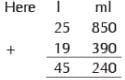Olympiad Test: Measurement - 1

# Olympiad Test: Measurement - 1

Test Description

## 10 Questions MCQ Test Math Olympiad for Class 5 | Olympiad Test: Measurement - 1

Olympiad Test: Measurement - 1 for Class 5 2022 is part of Math Olympiad for Class 5 preparation. The Olympiad Test: Measurement - 1 questions and answers have been prepared according to the Class 5 exam syllabus.The Olympiad Test: Measurement - 1 MCQs are made for Class 5 2022 Exam. Find important definitions, questions, notes, meanings, examples, exercises, MCQs and online tests for Olympiad Test: Measurement - 1 below.
Solutions of Olympiad Test: Measurement - 1 questions in English are available as part of our Math Olympiad for Class 5 for Class 5 & Olympiad Test: Measurement - 1 solutions in Hindi for Math Olympiad for Class 5 course. Download more important topics, notes, lectures and mock test series for Class 5 Exam by signing up for free. Attempt Olympiad Test: Measurement - 1 | 10 questions in 20 minutes | Mock test for Class 5 preparation | Free important questions MCQ to study Math Olympiad for Class 5 for Class 5 Exam | Download free PDF with solutions
 1 Crore+ students have signed up on EduRev. Have you?
Olympiad Test: Measurement - 1 - Question 1

### Shraddha has written following statements about the metric unit she would use to measure some objects. Find the incorrect sentence among them: 1. Centimetre is used to measure the length of a pencil. 2. Kilometre is used to measure distance from any city to another. 3. Metre is used to measure depth of a bucket. 4. Metre is used to measure height of a tree.

Olympiad Test: Measurement - 1 - Question 2

### A carpenter was putting up a shelf. Shelf needed to be 86 cm long but piece of wood he had was 1 m and 26 cm long. His saw was 33 cm long. How much did he have to cut off the piece of wood to make it fit?

Detailed Solution for Olympiad Test: Measurement - 1 - Question 2

The length of the wood = 1 m and 26 cm

= 126 cm.

Length of shelf = 86 cm.

Length he needs to cut off = 126 cm - 86 cm

= 40 cm.

Olympiad Test: Measurement - 1 - Question 3

### If 1 metre : 100 centimetre : : then 1 kilometre : ?

Detailed Solution for Olympiad Test: Measurement - 1 - Question 3

We know 1 kilometre = 1000 metre.

Olympiad Test: Measurement - 1 - Question 4

Direction: Add the following capacities:

25 l 850 ml and 19 l 390 ml.

Detailed Solution for Olympiad Test: Measurement - 1 - Question 4Olympiad Test: Measurement - 1 - Question 5

Direction: Add the following capacities:

17 l 708 ml and 13 l 993 ml

Olympiad Test: Measurement - 1 - Question 6

Direction: Add the following capacities:

75 l 95 ml and 12 l 938 ml

Olympiad Test: Measurement - 1 - Question 7

A container has 2550 ml of water. How many litres and millilitres of water is in the container?

Detailed Solution for Olympiad Test: Measurement - 1 - Question 7

We have 2550 ml = (2000 + 550) ml
= 2000 ml + 550 ml
= 2 l 550 ml

Olympiad Test: Measurement - 1 - Question 8

Rohan bought a 50 lt container of oil. He used 44 litres 300 ml of it. How much oil is left?

Olympiad Test: Measurement - 1 - Question 9

A jar can hold 4 l 250 ml honey. How much honey will be needed to fill 4 jars?

Detailed Solution for Olympiad Test: Measurement - 1 - Question 9

Required money = 4 × (4 l + 250) ml
= 16 l + 1000 ml
= 16 l + 1 l = 17 l

Olympiad Test: Measurement - 1 - Question 10

Shraddha needs 6 containers which can hold 15 l 600 ml oil. Find the capacity of each container.

## Math Olympiad for Class 5

2 videos|3 docs|40 tests
 Use Code STAYHOME200 and get INR 200 additional OFF Use Coupon Code
Information about Olympiad Test: Measurement - 1 Page
In this test you can find the Exam questions for Olympiad Test: Measurement - 1 solved & explained in the simplest way possible. Besides giving Questions and answers for Olympiad Test: Measurement - 1, EduRev gives you an ample number of Online tests for practice

## Math Olympiad for Class 5

2 videos|3 docs|40 tests